# Bessel polynomials

Jump to: navigation, search

Related to Bessel functions, [a2], the Bessel polynomials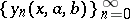satisfyand are given byThe ordinary Bessel polynomials are those found with, [a2].

The moments associated with the Bessel polynomials satisfy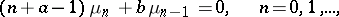and are given by.

The weight equation is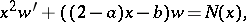whereis any function with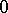moments. This equation has been solved when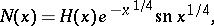wherewhen(no restriction),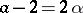and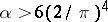, [a3]. The weight for the ordinary Bessel polynomials was found by S.S. Kim, K.H. Kwon and S.S. Han, [a1], after over 40 years of search.

Using the three-term recurrence relation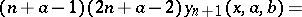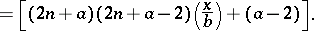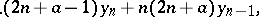the norm square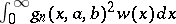is easily calculated and equals, [a2], where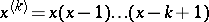. Clearly,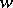generates a Krein space on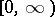.